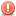##doubly linked list insert HElP

i need help with the insert method, i can't get it working if the timestamp is lesser than the current

public class DoubleList
{
private DoubleNode tail;

public DoubleList()
{
tail = null;
}

public boolean isEmpty()
{
}

{
}

{
if (isEmpty())
{
node.setPrev(null);
node.setNext(null);
tail = node;
}
else
{
node.setPrev(null);
}
}

public void insertTail(DoubleNode node)
{
if (isEmpty())
{
node.setNext(null);
node.setPrev(null);
tail = node;
}
else
{
tail.setNext(node);
node.setNext(null);
node.setPrev(tail);
tail = node;
}
}

{
{
tail = null;
}
else
{
}
}

public void deleteTail()
{
{
tail = null;
}
else
{
tail.getPrev().setNext(null);
tail = tail.getPrev();
}
}

public boolean insert(Event event)
{
DoubleNode newNode = new DoubleNode(event);
String timestamp = event.getTimestamp();
boolean result = false;

while (current != null)
{
if (timestamp.compareTo(current.getEvent().getTimestamp()) < 0)
{
{
}
else if (current == tail)
{
insertTail(newNode);
}
else
{
// insert the new node before the current node
newNode.setNext(current);
newNode.setPrev(current.getPrev());
current.getPrev().setNext(newNode);
current.setPrev(newNode);
}
result = true;
}
else
{
current = current.getNext();
}
}

if (current == null)
{
insertTail(newNode);
result = true;
}

return result;
}

public boolean delete(Event event)
{
boolean result = false;
String timestamp = event.getTimestamp();

while (current != null)
{
if (timestamp.compareTo(current.getEvent().getTimestamp()) == 0)
{
// remove the node
result = true;
}
else
{
current = current.getNext();
}
}
return result;
}
}